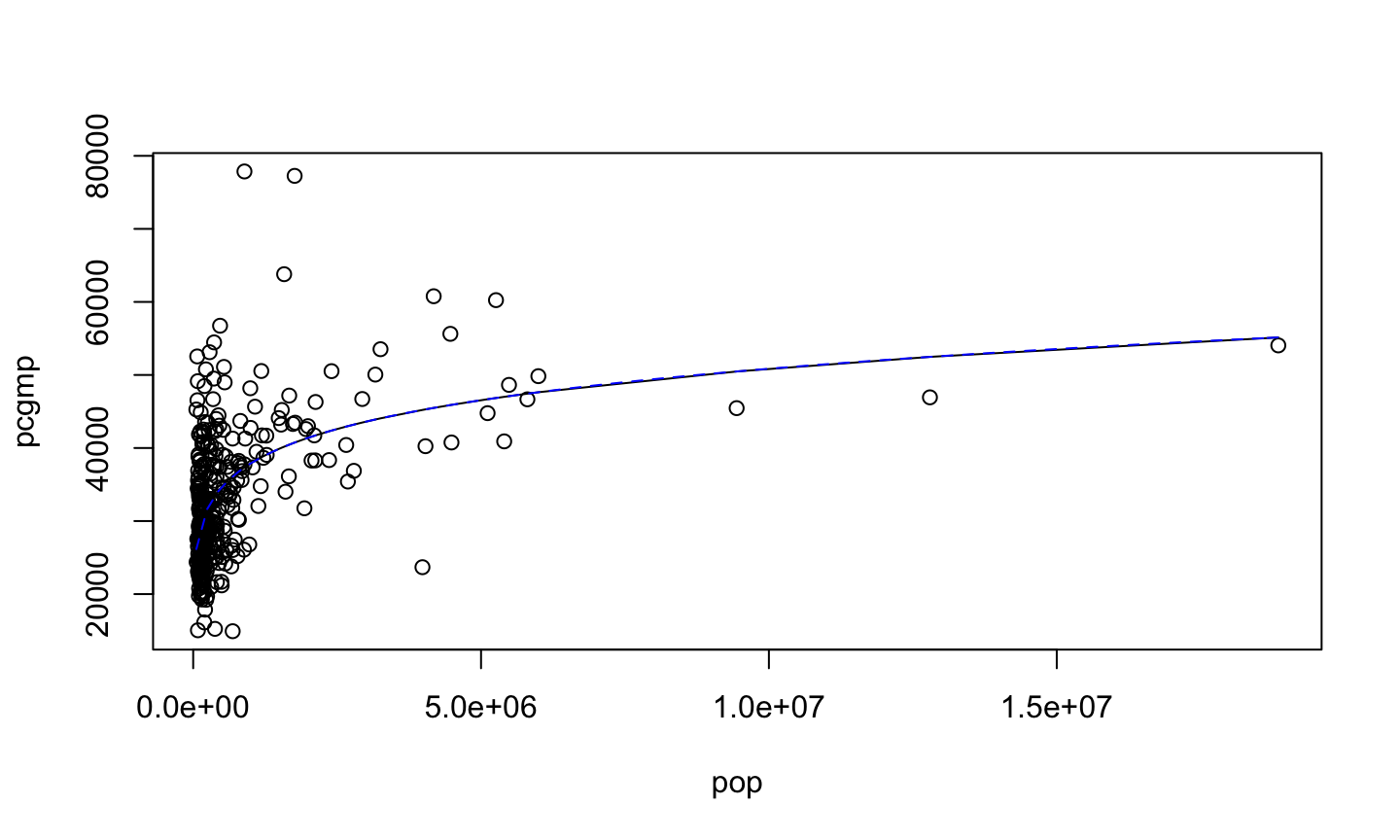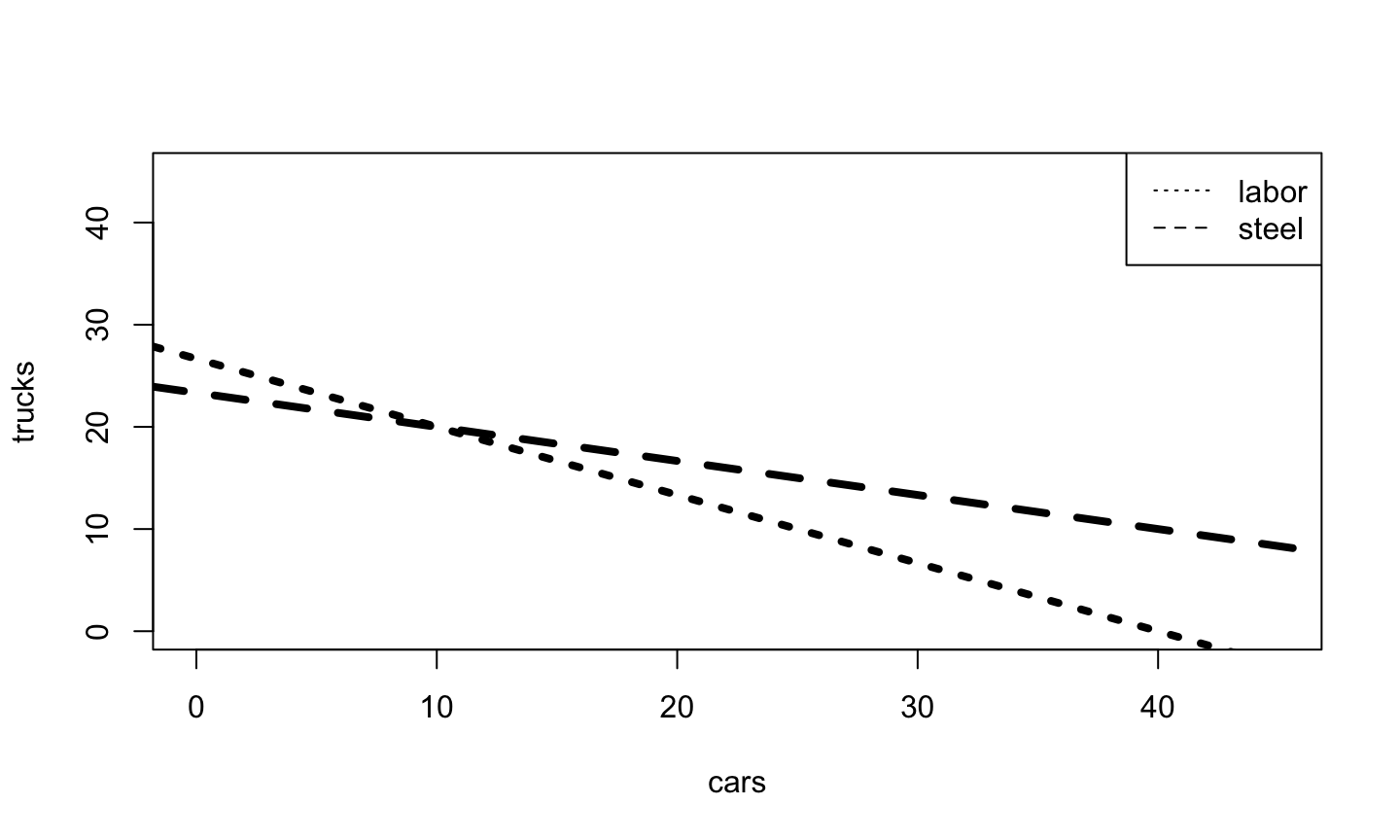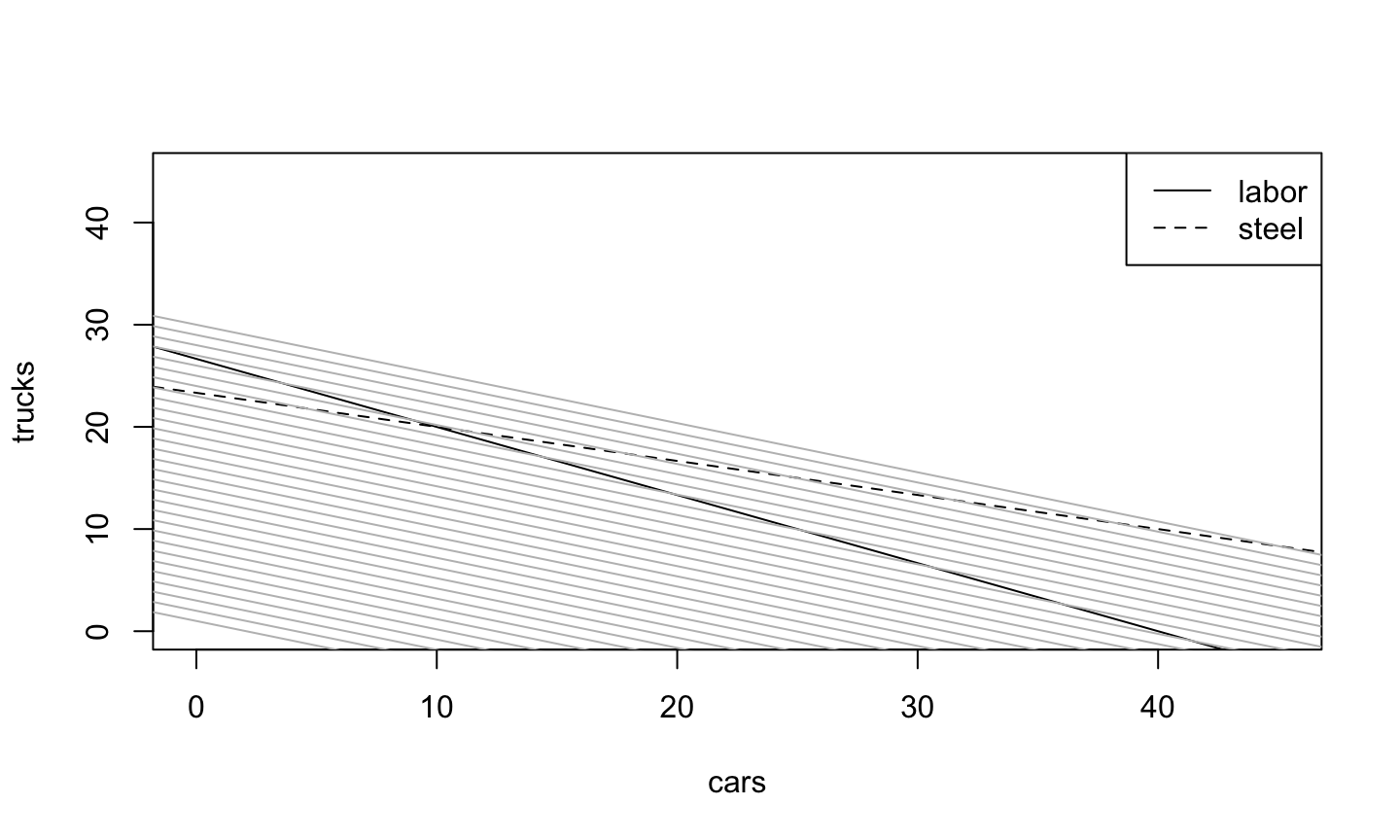## Agenda

• Optimization with optim() and nls()
• Optimization under constraints
• Lagrange multipliers
• Penalized optimization
• Statistical uses of penalized optimization

## Optimization in R: optim()

optim(par, fn, gr, method, control, hessian)

• fn: function to be minimized; mandatory
• par: initial parameter guess; mandatory
• gr: gradient function; only needed for some methods
• method: defaults to a gradient-free method (Nedler-Mead''), could be BFGS (Newton-ish)
• control: optional list of control settings
• (maximum iterations, scaling, tolerance for convergence, etc.)
• hessian: should the final Hessian be returned? default FALSE

Return contains the location ($par) and the value ($val) of the optimum, diagnostics, possibly $hessian ## Optimization in R: optim() gmp <- read.table("gmp.dat") gmp$pop <- gmp$gmp/gmp$pcgmp
library(numDeriv)
mse <- function(theta) {
mean((gmp$pcgmp - theta*gmp$pop^theta)^2)
}
theta0=c(5000,0.15)
fit1 <- optim(theta0,mse,grad.mse,method="BFGS",hessian=TRUE) 

## Optimization in R: optim()

fit1: Newton-ish BFGS method

fit1[1:3]
## $par ##  6493.2563739 0.1276921 ## ##$value
##  61853983
##
## $counts ## function gradient ## 63 11 ## Optimization in R: optim() fit1: Newton-ish BFGS method fit1[4:6] ##$convergence
##  0
##
## $message ## NULL ## ##$hessian
##              [,1]         [,2]
## [1,]      52.5021      4422071
## [2,] 4422071.3594 375729087979

## Optimization in R: nls()

• optim is a general-purpose optimizer
• nlm is another general-purpose optimizer; nonlinear least squares
• Try them both if one doesn't work

## Optimization in R: nls()

nls(formula, data, start, control, [[many other options]])
• formula: Mathematical expression with response variable, predictor variable(s), and unknown parameter(s)
• data: Data frame with variable names matching formula
• start: Guess at parameters (optional)
• control: Like with optim (optional)

Returns an nls object, with fitted values, prediction methods, etc. The default optimization is a version of Newton's method.

## Optimization in R: nls()

fit2: Fitting the Same Model with nls()

fit2 <- nls(pcgmp~y0*pop^a,data=gmp,start=list(y0=5000,a=0.1))
summary(fit2) 
##
## Formula: pcgmp ~ y0 * pop^a
##
## Parameters:
##     Estimate Std. Error t value Pr(>|t|)
## y0 6.494e+03  8.565e+02   7.582 2.87e-13 ***
## a  1.277e-01  1.012e-02  12.612  < 2e-16 ***
## ---
## Signif. codes:  0 '***' 0.001 '**' 0.01 '*' 0.05 '.' 0.1 ' ' 1
##
## Residual standard error: 7886 on 364 degrees of freedom
##
## Number of iterations to convergence: 5
## Achieved convergence tolerance: 1.819e-07

## Optimization in R: nls()

fit2: Fitting the Same Model with nls()

plot(pcgmp~pop,data=gmp)
pop.order <- order(gmp$pop) lines(gmp$pop[pop.order],fitted(fit2)[pop.order])
curve(fit1$par*x^fit1$par,add=TRUE,lty="dashed",col="blue")## Example: Multinomial

Roll dice $$n$$ times; $$n_1, \ldots n_6$$ count the outcomes

Likelihood and log-likelihood:

$\begin{eqnarray*} L(\theta_1,\theta_2,\theta_3,\theta_4,\theta_5,\theta_6) & = & \frac{n!}{n_1! n_2! n_3! n_4! n_5! n_6!}\prod_{i=1}^{6}{\theta_i^{n_i}}\\ \ell(\theta_1,\theta_2,\theta_3,\theta_4,\theta_5,\theta_6) & = & \log{\frac{n!}{n_1! n_2! n_3! n_4! n_5! n_6!}} + \sum_{i=1}^{6}{n_i\log{\theta_i}} \end{eqnarray*}$

Optimize by taking the derivative and setting to zero:

$\begin{eqnarray*} \frac{\partial \ell}{\partial \theta_1} & = & \frac{n_1}{\theta_1} = 0\\ \therefore \theta_1 & =& \infty \end{eqnarray*}$

## Example: Multinomial

We forgot that $$\sum_{i=1}^{6}{\theta_i}=1$$

We could use the constraint to eliminate one of the variables $\theta_6 = 1 - \sum_{i=1}^{5}{\theta_i}$

Then solve the equations $\frac{\partial \ell}{\partial \theta_i} = \frac{n_1}{\theta_i} -\frac{n_6}{1-\sum_{j=1}^{5}{\theta_j}} = 0$

BUT eliminating a variable with the constraint is usually messy

## Lagrange multipliers

$g(\theta) = c ~ \Leftrightarrow ~ g(\theta)-c=0$

Lagrangian:

$\mathcal{L}(\theta,\lambda) = f(\theta) - \lambda(g(\theta)-c)$

$$= f$$ when the constraint is satisfied

Now do unconstrained minimization over $$\theta$$ and $$\lambda$$:

$\begin{eqnarray*} {\left.\nabla_{\theta}\mathcal{L}\right|}_{\theta^*,\lambda^*} & = & \nabla f(\theta^*) - \lambda^*\nabla g(\theta^*) =0\\ {\left. \frac{\partial \mathcal{L}}{\partial \lambda}\right|}_{\theta^*,\lambda^*} & = & g(\theta^*) - c = 0 \end{eqnarray*}$

optimizing Lagrange multiplier $$\lambda$$ enforces constraint

More constraints, more multipliers

## Lagrange multipliers

Try the dice again:

$\begin{eqnarray*} \mathcal{L} & = & \log{\frac{n!}{\prod_i{n_i!}}} + \sum_{i=1}^{6}{n_i\log{(\theta_i)}} - \lambda\left(\sum_{i=1}^{6}{\theta_i} - 1\right)\\ {\left.\frac{\partial\mathcal{L}}{\partial \theta_i}\right|}_{\theta_i=\theta^*_i} & = & \frac{n_i}{\theta^*_i} - \lambda^* = 0\\ \frac{n_i}{\lambda^*} & = & \theta^*_i\\ \sum_{i=1}^{6}{\frac{n_i}{\lambda^*}} & = & \sum_{i=1}^{6}{\theta^*_i} = 1\\ \lambda^* & =& \sum_{i=1}^{6}{n_i} ~ \Rightarrow \theta^*_i = \frac{n_i}{\sum_{i=1}^{6}{n_i}} \end{eqnarray*}$

## Lagrange multipliers

Constrained minimum value is generally higher than the unconstrained

Changing the constraint level $$c$$ changes $$\theta^*$$, $$f(\theta^*)$$

$\begin{eqnarray*} \frac{\partial f(\theta^*)}{\partial c} & = & \frac{\partial \mathcal{L}(\theta^*,\lambda^*)}{\partial c}\\ & = & \left[\nabla f(\theta^*)-\lambda^*\nabla g(\theta^*)\right]\frac{\partial \theta^*}{\partial c} - \left[g(\theta^*)-c\right]\frac{\partial \lambda^*}{\partial c} + \lambda^* = \lambda^* \end{eqnarray*}$

$$\lambda^* =$$ Rate of change in optimal value as the constraint is relaxed

$$\lambda^* =$$ Shadow price'': How much would you pay for minute change in the level of the constraint

## Inequality Constraints

$h(\theta) \leq d ~ \Leftrightarrow ~ h(\theta) - d \leq 0$

The region where the constraint is satisfied is the feasible set

Roughly two cases:

1. Unconstrained optimum is inside the feasible set $$\Rightarrow$$ constraint is inactive
2. Optimum is outside feasible set; constraint is active, binds or bites; constrained optimum is usually on the boundary

Add a Lagrange multiplier; $$\lambda \neq 0$$ $$\Leftrightarrow$$ constraint binds

## Mathematical Programming

Older than computer programming…

Optimize $$f(\theta)$$ subject to $$g(\theta) = c$$ and $$h(\theta) \leq d$$

Give us the best deal on $$f$$, keeping in mind that we've only got $$d$$ to spend, and the books have to balance''

Linear programming

1. $$f$$, $$h$$ both linear in $$\theta$$
2. $$\theta^*$$ always at a corner of the feasible set

## Example: Factory

Revenue: 13k per car, 27k per truck

Constraints:

$\begin{eqnarray*} 40 * \mathrm{cars} + 60*\mathrm{trucks} & < & 1600 \mathrm{hours} \\ 1 * \mathrm{cars}+ 3 * \mathrm{trucks} & < & 70 \mathrm{tons} \end{eqnarray*}$

Find the revenue-maximizing number of cars and trucks to produce

## Example: Factory

The feasible region:## Example: Factory

The feasible region, plus lines of equal profit## More Complex Financial Problem

Given: expected returns $$r_1, \ldots r_p$$ among $$p$$ financial assets, their $$p\times p$$ matrix of variances and covariances $$\Sigma$$

Find: the portfolio shares $$\theta_1, \ldots \theta_n$$ which maximizes expected returns

Such that: total variance is below some limit, covariances with specific other stocks or portfolios are below some limit
e.g., pension fund should not be too correlated with parent company

Expected returns $$f(\theta) = r\cdot\theta$$

Constraints: $$\sum_{i=1}^{p}{\theta_i}=1$$, $$\theta_i \geq 0$$ (unless you can short)
Covariance constraints are linear in $$\theta$$
Variance constraint is quadratic, over-all variance is $$\theta^T \Sigma \theta$$

## Barrier Methods

(a.k.a. "interior point", "central path", etc.)

Having constraints switch on and off abruptly is annoying, especially with gradient methods

Fix $$\mu >0$$ and try minimizing $f(\theta) - \mu\log{\left(d-h(\theta)\right)}$ "pushes away" from the barrier — more and more weakly as $$\mu \rightarrow 0$$

## Barrier Methods

1. Initial $$\theta$$ in feasible set, initial $$\mu$$
2. While ((not too tired) and (making adequate progress))
1. Minimize $$f(\theta) - \mu\log{\left(d-h(\theta)\right)}$$
2. Reduce $$\mu$$
3. Return final $$\theta$$

## R implementation

constrOptim implements the barrier method

Try this:

factory <- matrix(c(40,1,60,3),nrow=2,
dimnames=list(c("labor","steel"),c("car","truck")))
available <- c(1600,70); names(available) <- rownames(factory)
prices <- c(car=13,truck=27)
revenue <- function(output) { return(-output %*% prices) }
plan\$par
##   9.999896 20.000035

constrOptim only works with constraints like $$\mathbf{u}\theta \geq c$$, so minus signs

## Constraints vs. Penalties

$\DeclareMathOperator*{\argmax}{argmax} \DeclareMathOperator*{\argmin}{argmin} \argmin_{\theta : h(\theta) \leq d}{f(\theta)} ~ \Leftrightarrow \argmin_{\theta,\lambda}{f(\theta) - \lambda(h(\theta)-d)}$

$$d$$ doesn't matter for doing the second minimization over $$\theta$$

We could just as well minimize

$f(\theta) - \lambda h(\theta)$

Constrained optimization Penalized optimization
Constraint level $$d$$ Penalty factor $$\lambda$$

## Statistical applications of penalization

Mostly you've seen unpenalized estimates (least squares, maximum likelihood)

Lots of modern advanced methods rely on penalties

• For when the direct estimate is too unstable
• For handling high-dimensional cases
• For handling non-parametrics

## Ordinary least squares

No penalization; minimize MSE of linear function $$\beta \cdot x$$:

$\hat{\beta} = \argmin_{\beta}{\frac{1}{n}\sum_{i=1}^{n}{(y_i - \beta\cdot x_i)^2}} = \argmin_{\beta}{MSE(\beta)}$

Closed-form solution if we can invert matrices:

$\hat{\beta} = (\mathbf{x}^T\mathbf{x})^{-1}\mathbf{x}^T\mathbf{y}$

where $$\mathbf{x}$$ is the $$n\times p$$ matrix of $$x$$ vectors, and $$\mathbf{y}$$ is the $$n\times 1$$ matrix of $$y$$ values.

## Ridge regression

Now put a penalty on the magnitude of the coefficient vector: $\tilde{\beta} = \argmin_{\beta}{MSE(\beta) + \mu \sum_{j=1}^{p}{\beta_j^2}} = \argmin_{\beta}{MSE(\beta) + \mu \|\beta\|_2^2}$

Penalizing $$\beta$$ this way makes the estimate more stable; especially useful for

• Lots of noise
• Collinear data ($$\mathbf{x}$$ not of "full rank")
• High-dimensional, $$p > n$$ data (which implies collinearity)

This is called ridge regression, or Tikhonov regularization

Closed form: $\tilde{\beta} = (\mathbf{x}^T\mathbf{x} + \mu I)^{-1}\mathbf{x}^T\mathbf{y}$

## The Lasso

Put a penalty on the sum of coefficient's absolute values: $\beta^{\dagger} = \argmin_{\beta}{MSE(\beta) + \lambda \sum_{j=1}^{p}{|\beta_j|}} = \argmin_{\beta}{MSE(\beta) + \lambda\|\beta\|_1}$

This is called the lasso

• Also stabilizes (like ridge)
• Also handles high-dimensional data (like ridge)
• Enforces sparsity: it likes to drive small coefficients exactly to 0

No closed form, but very efficient interior-point algorithms (e.g., lars package)

## Spline smoothing

"Spline smoothing": minimize MSE of a smooth, nonlinear function, plus a penalty on curvature:

$\hat{f} = \argmin_{f}{\frac{1}{n}\sum_{i=1}^{n}{(y_i-f(x_i))^2} + \int{(f^{\prime\prime}(x))^2 dx}}$

This fits smooth regressions without assuming any specific functional form

• Lets you check linear models
• Makes you wonder why you bother with linear models

Many different R implementations, starting with smooth.spline

## How Big a Penalty?

Rarely know the constraint level or the penalty factor $$\lambda$$ from on high

Lots of ways of picking, but often cross-validation works well

• Divide the data into parts
• For each value of $$\lambda$$, estimate the model on one part of the data
• See how well the models fit the other part of the data
• Use the $$\lambda$$ which extrapolates best on average

## Summary

• Start with pre-built code like optim or nls, implement your own as needed
• Use Lagrange multipliers to turn constrained optimization problems into unconstrained but penalized ones
• Optimal multiplier values are the prices we'd pay to weaken the constraints
• The nature of the penalty term reflects the sort of constraint we put on the problem
• Shrinkage
• Sparsity
• Smoothness

## Example: Lasso

x <- matrix(rnorm(200),nrow=100)
y <- (x %*% c(2,1))+ rnorm(100,sd=0.05)
mse <- function(b1,b2) {mean((y- x %*% c(b1,b2))^2)}
coef.seq <- seq(from=-1,to=5,length.out=200)
m <- outer(coef.seq,coef.seq,Vectorize(mse))
l1 <- function(b1,b2) {abs(b1)+abs(b2)}
l1.levels <- outer(coef.seq,coef.seq,l1)
ols.coefs <- coefficients(lm(y~0+x))

## Example: Lasso

contour(x=coef.seq,y=coef.seq,z=m,drawlabels=FALSE,nlevels=30,col="grey",
main="Contours of MSE vs. Contours of L1")
points(0,0)